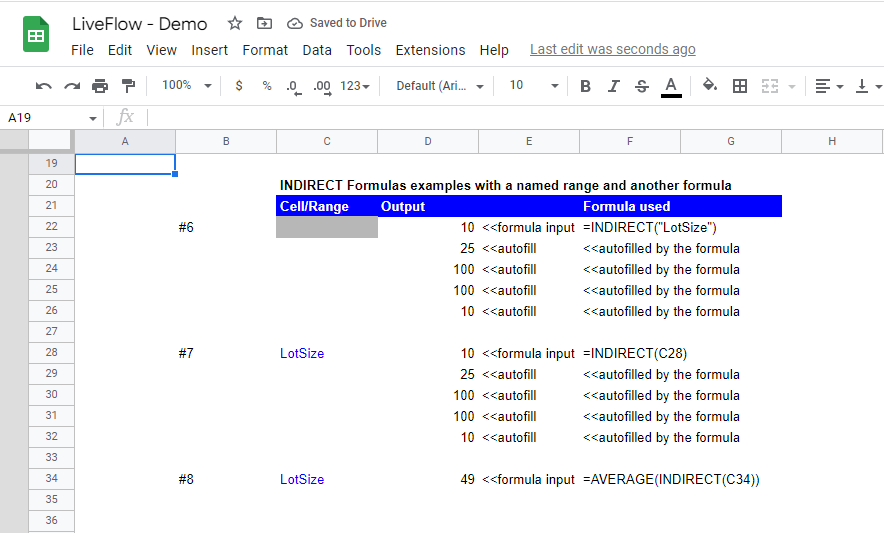# INDIRECT Function in Google Sheets: Explained

In this article, you will learn what the INDIRECT formula is and how to use it in Google Sheets.

## What does the INDIRECT formula do in Google Sheets?

The formula returns a value(s) in a cell or range specified in the formula. For example, if you type in “=INDIRECT(A2)”, it returns a value in cell A2. If you insert “=INDIRECT(A2:A4)”, it calls the values in the particular range. You can use this formula as a selected range, too. For insurance, you can create a formula like “=AVERAGE(INDIRECT(A2:A4))”, which gives you the average of the values in the range of cells A2 to A4, though you don’t necessarily do this in this simple case. Lastly, you can use Named Ranges in the formula to show the range or refer to it in another formula, as shown in the example above (the INDIRECT function used in the AVERAGE formula). In summary, this function is beneficial when you want to switch the cell or range referred to by just typing in the cell indexes, not by selecting them manually.

## How to use the INDIRECT function in Google Sheets

1. Type “=INDIRECT(” or navigate to the “Insert” tab (or “Functions” icon) → “Function”“Lookup”“INDIRECT”.
2. Insert a cell or range referred by cell reference or manual input with quotation marks such as “B2”.
3. Press the “Enter” key.

The general syntax is as follows:

``=INDIRECT(cell_reference_as_string, [is_A1_notation])``

Cell_reference_as_string: You can type in a cell or range’s indexes manually or use a cell reference in which you refer to a cell containing a cell or range’s indexes. When you use a cell reference, you don’t need to put quotation marks to the value in the referred cell. In case of manual input, don’t forget to enclose it with quotation marks.

Is_A1_notation (Optional): In this argument, you can insert “TRUE” or “FALSE” or keep it blank. If it’s blank, it is considered “TRUE” by default. This parameter is for the formula to recognize the cell reference properly. If it is “TRUE”, the function finds it the “A1” method of input, and if it is “FALSE”, it considers it the “R1C1” type of input. (A1 is equivalent to R1C1, meaning Row1Column1).

Note: If you input a range in “cell_reference_as_string”, the formula outputs the range automatically, so ensure you give enough space for the function to spread the result.

Look at the basic examples below to understand the formula and how to use it better. Note the “is_A1_notation” argument is blank in all INDIRECT functions in this article,

Examples #1 to 3 show how to call a value in a cell or a range on the same sheet.

## How to refer to a cell or range in a different worksheet in the same file

Look at the following two examples below.

Examples #4 and 5: These two cases present examples of pulling data from another sheet in the same file, filling the first argument by manual input (#4) or cell reference (#5). ‘62’!B5:C5 means a range of B5:C5 on the sheet named 62. The structure is ‘sheet name’ (sheet name enclosed by apostrophes), and a range is connected with !, an exclamation mark - ‘sheet name’+!+(a cell / a range). Also, even when you refer to another worksheet, you need to add quotation marks in case of manual input.

## How to use the INDIRECT function with Named Ranges or other functions

Look at the following three examples.How to use the INDIRECT formula with the Named Ranges or other functions

Examples #6 and #7 show how you can use named ranges in the INDIRECT formula. “LotSize” represents a range of D4:D8 on the sheet. Note you need to add quotation marks when you manually input the range's name. As you can see, the formula return values across the cells in the same direction as the original range.

Examples #8: This is the example of combining the INDIRECT function with another formula and Named Range. When you use the INDIRECT formula in another formula as a range, you don’t need to put quotation marks at the beginning and the end of the INDIRECT function.

## How do you use indirect validation in Google Sheets?

Check this article: Dependent Drop-down List in Google Sheets: Explained if you are looking for a way to create a dependent drop-down list whose choices change based on your choice in the first pull-down menu.

Learn how to do this step-by-step in the video below 👇

## Need help?

Our team is here to help you any time between 9am and 10pm EST.Email us at: help@liveflow.io